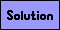Square Tiling Puzzles

All of the following problems involve tiling a square or rectangle with integer-sided squares.

1. Tile a square of side 37 with 15 squares.2. Tile a square of side 61 with squares, each having side at least 10.3. Tile a square of side 19 with squares, using each size square no more than twice.4. Tile a square of side 18 with squares, so that no two squares of the same size touch each other.5. Tile a square of side 29 with squares, so that each square not on the bottom lies on top of one or more larger squares.6. Tile a square of side 33 with squares of side 1 through 12, with each square being used either once or twice.7. Tile a square of side 16 with 22 squares so that every square touches a square of the next smallest size.8. Tile a square of side 36 with 1 square of side 1, 2 squares of side 2, . . . up to 8 squares of side 8.9. Tile a 33×35 rectangle with 3 squares of side 1, 3 squares of side 2, . . . up to 3 squares of side 10.10. Tile a 36×50 rectangle with 2 squares of side 2, 4 squares of side 4, . . . up to 10 squares of side 10.